# Numbers and Number System (Part - 2) Notes | Study Quantitative Techniques for CLAT - CLAT

## CLAT: Numbers and Number System (Part - 2) Notes | Study Quantitative Techniques for CLAT - CLAT

The document Numbers and Number System (Part - 2) Notes | Study Quantitative Techniques for CLAT - CLAT is a part of the CLAT Course Quantitative Techniques for CLAT.
All you need of CLAT at this link: CLAT

Division Algorithm

Division Algorithm is the arithmetic Identity and can be expressed as:

Dividend = Divisor x Quotient + Remainder

Or Quotient = (Dividend – Remainder) ÷ Divisor

Or Divisor = (Dividend – Remainder) ÷  Quotient

In long division,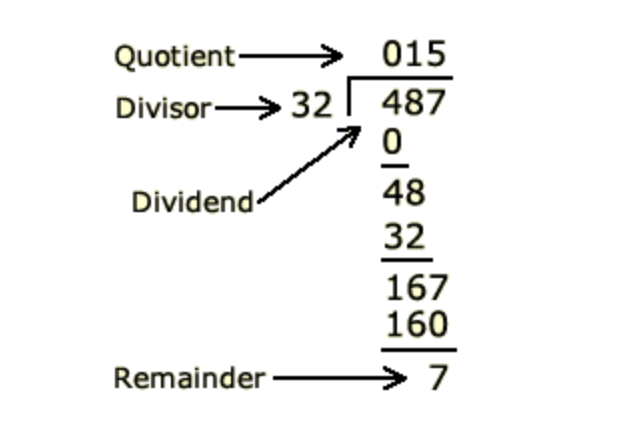Example of long division

Example 1. The quotient arising from the division of 23456 by a certain number is 89 and the remainder is 49. What is the divisor?

Solution: The division algorithm is:

Dividend = Divisor x Quotient + Remainder

Or Divisor = (Dividend – Remainder) ÷  Quotient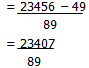= 263

So, divisor is 263.

Example 2: What least number must be subtracted from 5931 to make it exactly divisible by 94.

Solution: On dividing 5931 by 94 we get 9 as the remainder.

Therefore the number to be subtracted is 9.

Example 3: What least number must be added to 5931 to make it exactly divisible by 94.

Solution: On dividing 5931 by 94 we get 9 as the remainder. So the number to be added to 5931 to make it exactly divisible by 94 is 94-9=85

Note: When we are to find out the number to be subtracted as in example 2, we simply subtract the remainder, but when the least number to be added is to be found out, as in Example 3, we find out the difference of the divisor and the remainder and get the least number which can be added.

Example 4: Find the least number of five digits which is exactly divisible by 129.

Solution: The least number of five digits is 10,000. On dividing 10,000 by 129 we get 77 as the quotient and 67 as the remainder.

We can get the number exactly divisible by 129 by subtracting 67 from 10000, but in that case the number which we get will be of four digits only. But we are to find out the least number of five digits, so we have to add something in 10000. The number to be added can be found out by subtracting the remainder from the divisor.

So 129 – 67 = 62

So 62 is the number which should be added.

We get the answer as 10000 + 62 = 10062.

Example 5: Find the greatest number of five digits which is exactly divisible by 129.

Solution: The greatest number of five digits is 99,999. On dividing 99,999 by 129 we get 24 as the remainder.

So the required number is 99999 – 24 = 99975

Note: While finding the least no. we added the difference between the divisor and the remainder but for finding the greatest number we subtracted the remainder.

Example 6: A number when divided by 296 leaves remainder 75. What will be the remainder if same number is divided by 37.

Solution: In such questions check whether the first divisor (i.e. 296) is exactly divisible by the 2nd divisor. If so that the remainder left by dividing the given number by 1st divisor is to be divided by the 2nd divisor and we get the answer.

In this case the first divisor 296 is exactly divisible by the 2nd divisor i.e. 37. So we simply divide the remainder 75 by 2nd divisor (37) and the remainder so left will be our answer.

75 ÷  37 gives 2 as the quotient and 1 as the remainder. So 1 is the answer

Example 7: A number when divided by 114 leaves the remainder as 21. If the same number is divided by 19, then what will be the remainder.

Solution: First divisor (114) is exactly divisible by second divisor 19. Now the remainder 21 is to be divided by 19. It will give 2 as the answer.

Note: In this type of questions, the first divisor must be exactly divisible by the second divisor, otherwise the question is not possible.

Example 8: Find the number in the unit place in

1) (321)45
2) (322)57
3) (323)76
4) (324)41
5) (325)95
6) (326)91
7) (327)31
8) (328)43
9) (329)87

Solution: The method to solve such questions is illustrated below.

In the expression pn, p is called the base and n is called the power or exponent.

Solution for Sr. nos (i) , (v) and (vi)

If the base is having 1, 5 or 6 at the unit place, and it is raised to any power the number at the unit place of the result does not change.

For example (i) (321)1 will be 321 which has 1 at the unit place

(321)2 = 321 x 321 = a number which must have 1 at the unit place.

Similarly (321)3, (321)4 or 321 raised to any power say (321)45 will have 1 at the unit place of the result.

(v) (325)1 = 325 has 5 at the unit place.

(325)2 = 325 x 325 will have 5 at the unit place.

also (325)3 = 325 x 325 x 325 when unit place of these 3 factors is multiplied we get 125 which gives 5 at the unit place.

Similarly (325)4, (325)5 or (325)95 when expanded will give 5 at the unit place.

(vi) (326)1 = 326 has 6 at the unit place.

(326)= 326 x 326 when digit 6 at the unit place of these two factors is multiplied we get 36 which has 6 at the unit place.

Similarly 326 raised to any power i.e. 3, 4, 5 etc will give 6 at the unit place.

So (326)91 on expansion will have 6 at the unit place.

(ii) (322)1 = 322 has 2 at the unit place.

(322)2 = 322 x 322 has 4 at the unit place.

(322)3 = 322 x 322 x 322 has 8 at the unit place

(322)4 = 322 x 322 x 322 x 322 has 6 at the unit place.

(322)5 = 322 x 322 x 322 x 322 x 322 has 2 at the unit place.

It means we have got 2 at the unit place after every four iterations.

Let us rewrite

322 power 1 has 2 at the unit place.

322 power 2 has 4 at the unit place.

322 power 3 has 8 at the unit place.

322 power 4 has 6 at the unit place.

322 power 5 has 2 at the unit place.

So 1, 5, 9, 13, 17 etc. will give 2 at the unit place. Now let us consider (322)57

57/4  = 14 and remainder is 1.

So power 1 we know gives 2 at the unit place. So the digit at the unit place in (322)57 is 2

(iii) In (323)76

(323)1 = 323 so power 1 of 323 has 3 at unit place.

(323)2 = 323 x 323 so power 2 has 9 at unit place.

(323)3 = 323 x 323 x 323 so power 3 has 7 at the unit place.

(323)4 = 323 x 323 x 323 x 323, so power 4 has 1 at the unit place.

(323)5 = 323 x 323 x 323 x 323 x 323 so power 5 has 3 at the unit place.

So after adding 4 to the power 1, 3 has been repeated.

So naturally power 6 has 9, power 7 has 7, power 8 has 1 and power 9 has 3 at the unit place.

So on dividing the given power by 4,

If remainder is 1, digit at the unit place will be 3.

If remainder is 2, digit at the unit place will be 9.

If remainder is 3, digit at the unit place will be 7.

If remainder is 4, digit at the unit place will be 1 (remainder 4 means, the number is exactly divisible by 4).

(iv) (324)41

If power of 324 is 1, digit at the unit place is 4

If power of 324 is 2, digit at the unit place is 4 x 4 = 16 = 6

If power of 324 is 3, digit at the unit place is 6 x 4 = 24 i.e. 4

The unit place digit is either 4 or 6

If power is odd the digit at unit place is 4

If power is even the digit at unit place is 6

Since (324)41 the power is odd, so the answer is 4

(vii) (327)31

If power of 327 is 1, the digit at unit place is 7

If power of 327 is 2, the digit at unit place is 7 x 7 = 49 so 9

If power of 327 is 3, the digit at unit place is 9 x 7 = 63 so 3

If power of 327 is 4, the digit at unit place is 3 x 7 = 21 = 1

If power of 327 is 5, the digit at unit place is 1 x 7 = 7

At power 5 the digit at the unit place is the same as at the time of power 1

So divide the power 31 by 4, we get 3 as the remainder. But if power is 3 then the digit at unit place in 3.

(viii) (328)43

If power is 1, digit at unit place is 8

If power is 2, digit at unit place is 8 x 8 = 64 i.e. 4

If power is 3, digit at unit place is 4 x 8 = 32 i.e. 2

If power is 4, digit at unit place is 2 x 8 = 16 i.e. 6

If power is 5, digit at unit place is 6 x 8 = 48 i.e. 8

So after every gap of 4 the digit at unit place is being repeated.

So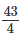gives 3 as remainder and therefore the digit at the unit place is 2.

(ix) (329)87

If power of 329 is 1, the digit at unit place is 9.

If power of 329 is 2, the digit at unit place is 9 x 9 = 81 i.e. 1

If power of 329 is 3, the digit at unit place is 1 x 9 = 9.

If power of 329 is 4, the digit at unit place is 9 x 9 = 81 i.e. 1

So if power is 1 (odd) digit is 9.

So if power is 2 (even) digit is 1.

In the present case power is 87 and 87 is odd so the digit at unit place will be 9.

Rules for counting nos.

Natural numbers are called counting numbers.

Rule 1

Sum of the first n counting numbers.

= ∑ n =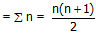Example: The sum of first 50 counting nos. is written as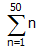and is equal to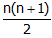where n = 50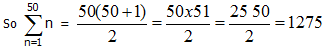Rule 2.

Sum of the first n odd numbers = n2 where n is the number of terms in the summation.

Example: To add first 25 odd numbers from 1 to 49 i.e. to find the sum of odd numbers

1 + 3 + 5 + 7 ------ + 49 (Here n = 25)

Sum = n2 = (25)2 = 625

Rule 3.

Sum of the first n even numbers = n(n + 1) where n is the number of terms in the summation.

Example: Find out the sum of the first 25 even numbers.

Solution: Sum = 2 + 4 + 6 + 8+........ 25 terms.

Here the no. of terms = 25 or n= 25

So Sum = n(n+1) = 25 (26) = 650

Note: Remember that the rules are true for first n numbers only.

Example: Find:

(1) The sum of first 100 counting numbers

(2) The sum of all odd nos. from 1 to 100

(3) The sum of all even nos. from 1 to 100

(4) The sum of all even nos. from 40 to 100

Solution: (1) Sum of first 100 counting nos.

Here n = 100.

So Sum =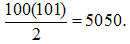(2) Sum of all odd numbers between 1 and 100

= 1 + 3 + 5 + 7+..........+ 99.

Here n = 50.

So sum = (n)2 = (50)2 = 2500

(3) Sum of all even numbers between 1 and 100

= 2 + 4 + 6 + 8+..........+ 100.

here n = 50

So sum = n (n + 1) = 50 (51) = 2550

(4) The rule cannot be applied here, as the numbers to be added lie between 40 and 100

We make the rule applicable by first adding all even numbers between 1 and 100 and then subtracting the sum of even numbers between 1 and 39.

So Sum of even numbers between 40 and 100 = Sum of even numbers between 1 and 100 – Sum of even nos. between 1 and 39.

Between 1 and 100, there are 50 terms of even nos. and between 1 and 39 there are 19 terms of even nos.

So Sum = 50 (50 + 1) ­­---- 19 (19 + 1)

= 50 x 51 ----- 19 x 20

= 25 50 – 380 = 2170

Rule 4.

Sum of the squares of first n natural numbers.

12 + 22 + 32 + ------- + n2 = ∑n2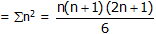Rule 5.

Sum of the cubes of first n natural numbers.

13 + 23 + 33 + -------- + n3 = ∑n3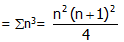Few algebraic Identities which help for solving arithmetic problems are:

(1) (a + b)2 = a2 + 2ab + b2

(2) (a – b)2 = a2 – 2ab + b2

(3) (a + b) (a – b) = a2 – b2

(4) (a + b)3 = a3 + b3 + 3ab (a + b)

(5) (a – b)3 = a3 – b3 – 3ab (a – b)

(6) a3 + b3 = (a + b) (a2 + b2 – ab) = (a + b)3 – 3ab (a + b)

(7) a3 – b3 = (a – b) (a2 + b2 + ab) = (a – b)3 + 3ab (a – b)

(8) a + b =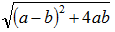(9) a – b =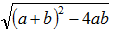The document Numbers and Number System (Part - 2) Notes | Study Quantitative Techniques for CLAT - CLAT is a part of the CLAT Course Quantitative Techniques for CLAT.
All you need of CLAT at this link: CLATUse Code STAYHOME200 and get INR 200 additional OFF

## Quantitative Techniques for CLAT

49 videos|23 docs|88 tests

Track your progress, build streaks, highlight & save important lessons and more!

,

,

,

,

,

,

,

,

,

,

,

,

,

,

,

,

,

,

,

,

,

;